# Civil Engineering - GATE Exam Questions

### Exercise :: GATE Exam Questions - Section 7

26.

The influence line diagram for the force in member 'a' of the truss shown below is given by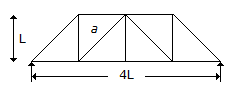A.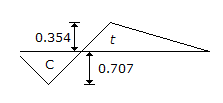B.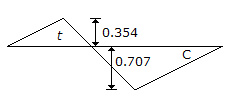C.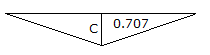D.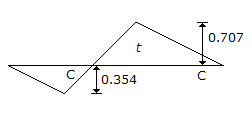Explanation:

No answer description available for this question. Let us discuss.

27.

In a BOD test using 5% dilution of the sample (15 ML of sample and 285 mL of dilution water), dissolved oxygen values for the sample and dilution water blank bottles after five days incubation at 20°C were 3.80 and 8.80 mg/L. respectively. Dissolved oxygen originally present in the undiluted sample was 0.80 mg/L. The 5-day 20°C BOD of the sample is

 A. 116 mg/L B. 108 mg/L C. 100 mg/L D. 92 Mg/L

Explanation:

No answer description available for this question. Let us discuss.

28.

Group symbols assigned to silty sand and clayey sand are respectively

 A. SS and CS B. SM and CS C. SM and SC D. MS and CS

Explanation:

No answer description available for this question. Let us discuss.

29.

The shape factor of the section shown in the below figure is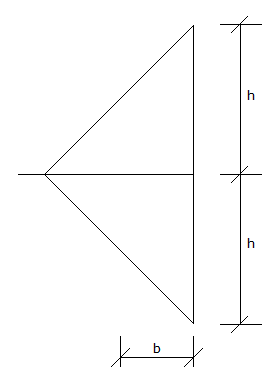A. 1.5 B. 1.12 C. 2 D. 1.7